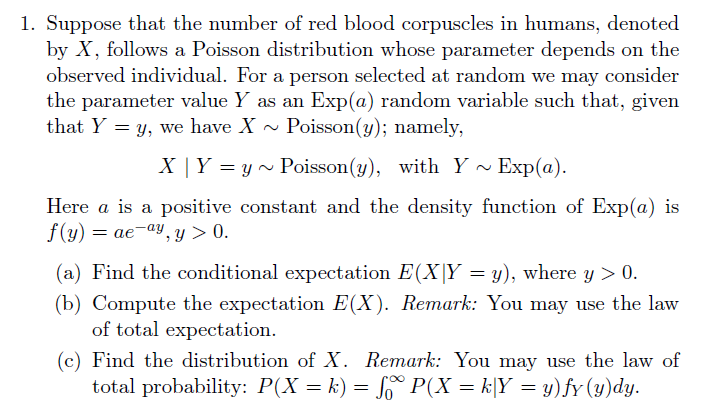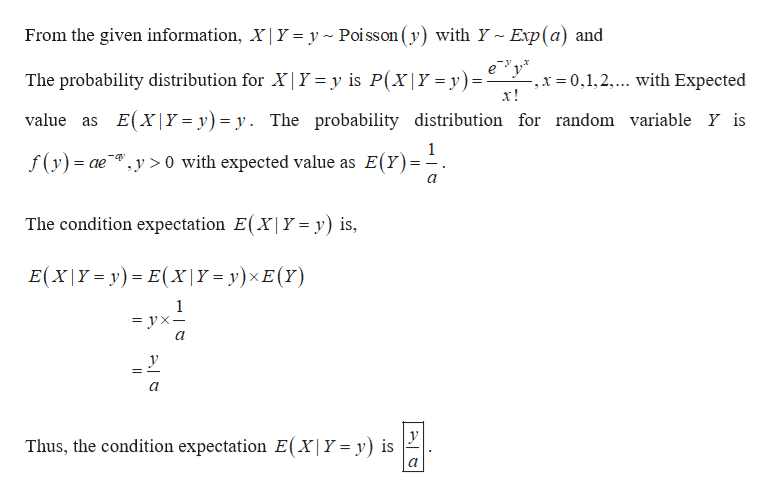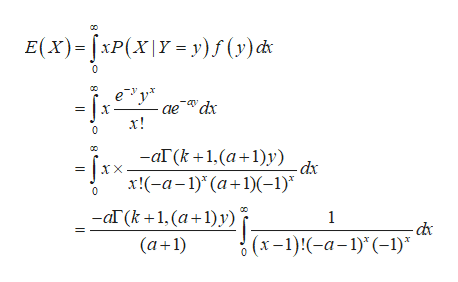1. Suppose that the number of red blood corpuscles in humans, denotedby X, follows a Poisson distribution whose parameter depends on theobserved individual. For a person selected at random we may considerthe parameter value Y as anthat Y y, we have XExp(a) random variable such that, givenPoisson(y); namely,Exp(a)X | Y yPoisson(y), with YHere a is apositive constant and the density function of Exp(a) isf(y)aeay, y > 0(a) Find the conditional expectation E(X|Y = y), where y 0(b) Compute the expectation E(X). Remark: You may use the lawof total expectation.(c) Find the distribution of X. Remark: You may use the law oftotal probability: P(X k) = So P(X k|Y y)fy (y)dy

Question

I need an easy to understand solution with a thorough explanation. I have no good background in probability. I hope you will provide me with a very good solution, Thank you very muchhelp_outlineImage Transcriptionclose1. Suppose that the number of red blood corpuscles in humans, denoted by X, follows a Poisson distribution whose parameter depends on the observed individual. For a person selected at random we may consider the parameter value Y as an that Y y, we have X Exp(a) random variable such that, given Poisson(y); namely, Exp(a) X | Y yPoisson(y), with Y Here a is a positive constant and the density function of Exp(a) is f(y)aeay, y > 0 (a) Find the conditional expectation E(X|Y = y), where y 0 (b) Compute the expectation E(X). Remark: You may use the law of total expectation. (c) Find the distribution of X. Remark: You may use the law of total probability: P(X k) = So P(X k|Y y)fy (y)dy fullscreen
Step 1

Find the condition expectationhelp_outlineImage TranscriptioncloseFrom the given information, X| Y = y Poi sson (y) with Y~ Exp(a) and ey The probability distribution for X|Y = y is P(X|Y = y) ,x 0,1,2.... with Expected х! = E(X|Y= y) = y. The probability distribution for random variable Y is value as f(y)ae >0 with expected value as E(Y) The condition expectation E(X| Y = y) is, E(X|Y-y) E(Xx|Y= y)xE(Y) 1 — ух— у Thus, the condition expectation E(X|Y = y) is fullscreen
Step 2

1.b)

Compute the Expectati...help_outlineImage TranscriptioncloseE(x)XP(xy y)f (y) c 0 ае dх х! 0 — аГ(k +1,(а+1)у) ir = Jxx-a-1) (a+1)(-1)" dx X 0 ar (k+1, (a+1)y) (a 1) 1 -dc (x-1)-a-1)(-1) fullscreen

Want to see the full answer?

See Solution

Want to see this answer and more?

Our solutions are written by experts, many with advanced degrees, and available 24/7

See Solution
Tagged in

Probability